Importance: Low ✭
 Author(s):
 Subject: Graph Theory » Coloring » » Vertex coloring
 Keywords: choosability complete multipartite graph list coloring
 Posted by: Jon Noel on: April 11th, 2013
 Solved by: H. A. Kierstead, A. Salmon and R. Wang
Question   What is the choice number offor general?

For positive integersand, let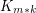be the complete-partite graph in which every part has size. For the definition of the choice number (list chromatic number) see the Wikipedia page.

Determining the choice number ofseems to be a natural first step to obtaining a general bound on the choice number of graphs on a bounded number of vertices.

An old result of Erdos, Rubin and Taylor [ERT80] is that the choice number ofis. Ohba [Ohb02] conjectured a generalization of this result which was proved by Noel, Reed and Wu [NRW12] (also see [Noe13]): if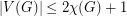, then the choice number ofis.

Kierstead [Kier00] proved that the choice number of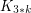is exactly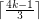. The upper bound was generalized by Noel, West, Wu and Zhu [NWWZ13] to the following: if, then the choice number ofis at most TeX Embedding failed!.}

More generally, Noel et al. [NWWZ13] proved that, for any graph, the choice number ofis at most(also see [Noe13]). This implies the following:

Theorem  (Noel et al. 2013)   The choice number ofis at most.

A lower bound on the choice number ofis given by Yang [Yan03]:

Theorem  (Yang 2003)   The choice number ofis at least.

The problem was recently solved by Kierstead, Salmon and Wang [KSW14]. They proved that, surprisingly, the lower boundis tight for all.

Theorem  (Kierstead, Salmon and Wang 2014)   The choice number ofis equal to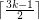.

Naturally, we wonder whether this result can be generalised to all graphs on at mostvertices. See this posting as well.

Question   Is it true that if, then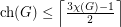?

Bibliography

[ERT80] P. Erdos, A. L. Rubin, and H. Taylor. Choosability in graphs. Congress. Numer., XXVI, pages 125–157, 1980.

[Kie00] H. A. Kierstead. On the choosability of complete multipartite graphs with part size three. Discrete Math., 211(1-3):255–259, 2000.

[KSW14] H. A. Kierstead, A. Salmon and R. Wang. On the Choice Number of Complete Multipartite Graphs With Part Size Four.

[Noe13] J. A. Noel. Choosability of Graphs With Bounded Order: Ohba's Conjecture and Beyond. Master's thesis, McGill University, Montreal. pdf

[NRW12] J. A. Noel, B. A. Reed, and H. Wu. A Proof of a Conjecture of Ohba. Preprint, arXiv:1211.1999v1, November 2012. Webpage

[NWWZ13] J. A. Noel, D. B. West, H. Wu, and X. Zhu. Beyond Ohba's Conjecture: A bound on the choice number of-chromatic graphs withvertices. Preprint, arXiv:1308.6739v1, August 2013. pdf

[Ohb02] K. Ohba. On chromatic-choosable graphs. J. Graph Theory, 40(2):130–135, 2002.

[Yan03] D. Yang. Extension of the game coloring number and some results on the choosability of complete multipartite graphs. PhD thesis, Arizona State University, Tempe, Arizona, 2003.

* indicates original appearance(s) of problem.